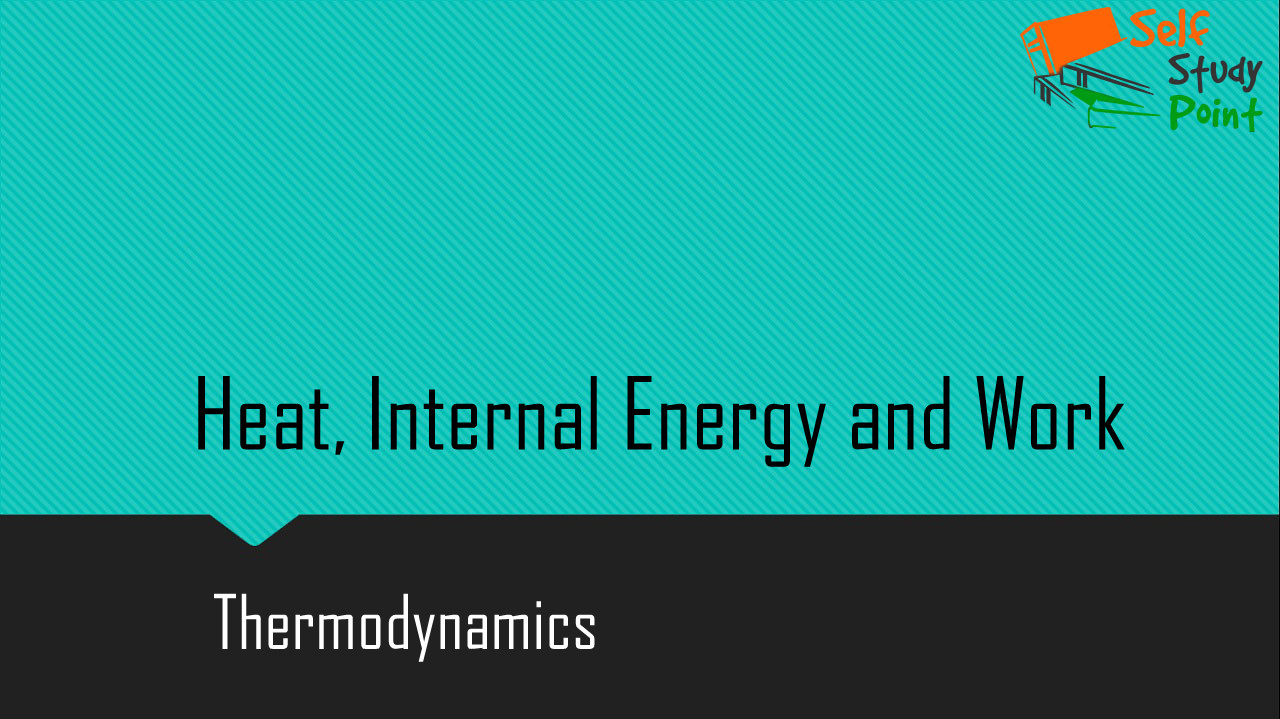## Heat and work:

Heat Work
Heat is a mode of energy transfer due to the temperature difference between the system and the surrounding. Work is the mode of energy transfer brought about by means that do not involve temperature difference.
When heat is supplied to a gas, its molecules move faster in all directions at random. So, heat is a mode of energy transfer that produces random motion When a piston compresses a gas to do work on it, it forces the molecules to move in the direction of piston’s motion. So work may be regarded as the mode of energy transfer that produces organized motion.

## Internal energy:

1. The internal energy of a system is the sum of molecular kinetic and potential energies in the frame of reference relative to which the center of mass of the system is at rest.
2. The intermolecular potential energy of a real gas is a function of its volume.
3. The internal kinetic energy of a gas is a function of its temperature.
4. The internal energy of a system is a thermodynamic state variable.
5. The internal energy of an ideal gas is purely kinetic in nature.

### Sign conventions used:

1. Heat absorbed by a system is positive. Heat given out by a system is negative.
2. Work done by a system is positive. Work done on a system is negative.
3. The increase in internal energy of a system is positive. The decrease in internal energy of a system is negative.

In thermodynamics, heat and work are not stable variables. These are the modes of energy transfer to a system resulting in the change in its internal energy. Thus the statement like ‘a gas in a given state has a certain amount of heat or work’ is meaningless; on the other hand, the statement like ‘a certain amount of heat is supplied to the system or a certain amount of work is done by the system’ is meaningful.

Click on a star to rate it!

Average rating / 5. Vote count:

No votes so far! Be the first to rate this post.